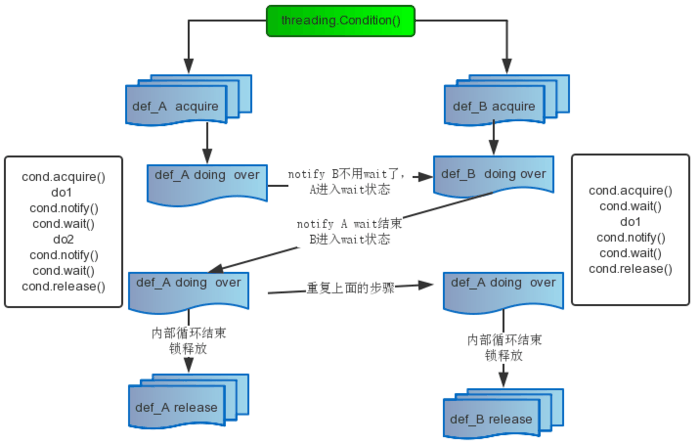# 多线程编程

• New 创建
• Runnable 就绪。等待调度
• Running 运行
• Blocked 阻塞。阻塞可能在 Wait Locked Sleeping
• Dead 消亡

• 主线程
• 子线程
• 守护线程（后台线程）
• 前台线程

## 1、线程的创建

Python 提供两个模块进行多线程的操作，分别是 `thread``threading`

``````#!/usr/bin/env python3
# -*- coding: UTF-8 -*-

import time
import threading

class MyThread(threading.Thread):
def run(self):
for i in range(5):
print('thread {}, @number: {}'.format(self.name, i))
time.sleep(1)

def main():
print("Start main threading")

# 创建三个线程
threads = [MyThread() for i in range(3)]
# 启动三个线程
for t in threads:
t.start()

print("End Main threading")

if __name__ == '__main__':
main()

``````

``````Start main threading
thread Thread-1, @number: 0
thread Thread-2, @number: 0
thread Thread-3, @number: 0
End Main threading
thread Thread-2, @number: 1
thread Thread-1, @number: 1
thread Thread-3, @number: 1
thread Thread-1, @number: 2
thread Thread-3, @number: 2
thread Thread-2, @number: 2
thread Thread-2, @number: 3
thread Thread-3, @number: 3
thread Thread-1, @number: 3
thread Thread-3, @number: 4
thread Thread-2, @number: 4
thread Thread-1, @number: 4
``````

## 2、线程合并（join方法）

``````#!/usr/bin/env python3
# -*- coding: UTF-8 -*-

import time
import threading

class MyThread(threading.Thread):
def run(self):
for i in range(5):
print('thread {}, @number: {}'.format(self.name, i))
time.sleep(1)

def main():
print("Start main threading")

# 创建三个线程
threads = [MyThread() for i in range(3)]
# 启动三个线程
for t in threads:
t.start()

# 一次让新创建的线程执行 join
for t in threads:
t.join()

print("End Main threading")

if __name__ == '__main__':
main()

``````

``````Start main threading
thread Thread-1, @number: 0
thread Thread-2, @number: 0
thread Thread-3, @number: 0
thread Thread-1, @number: 1
thread Thread-3, @number: 1
thread Thread-2, @number: 1
thread Thread-2, @number: 2
thread Thread-1, @number: 2
thread Thread-3, @number: 2
thread Thread-2, @number: 3
thread Thread-1, @number: 3
thread Thread-3, @number: 3
thread Thread-3, @number: 4
thread Thread-2, @number: 4
thread Thread-1, @number: 4
End Main threading

``````

## 3、线程同步与互斥锁

``````lock = threading.Lock()
``````

``````lock.acquire()
``````

``````lock.release()
``````

``````r_lock = threading.RLock()
``````

## 4、Condition 条件变量

Python 提供了 Condition 对象。``````#!/usr/bin/env python3
# -*- coding: UTF-8 -*-

import threading, time

class Consumer(threading.Thread):
def __init__(self, cond, name):
# 初始化
super(Consumer, self).__init__()
self.cond = cond
self.name = name

def run(self):
# 确保先运行Seeker中的方法
time.sleep(1)
self.cond.acquire()
print(self.name + ': 我这两件商品一起买，可以便宜点吗')
self.cond.notify()
self.cond.wait()
print(self.name + ': 我已经提交订单了，你修改下价格')
self.cond.notify()
self.cond.wait()
print(self.name + ': 收到，我支付成功了')
self.cond.notify()
self.cond.release()
print(self.name + ': 等待收货')

class Producer(threading.Thread):
def __init__(self, cond, name):
super(Producer, self).__init__()
self.cond = cond
self.name = name

def run(self):
self.cond.acquire()
# 释放对琐的占用，同时线程挂起在这里，直到被 notify 并重新占有琐。
self.cond.wait()
print(self.name + ': 可以的，你提交订单吧')
self.cond.notify()
self.cond.wait()
print(self.name + ': 好了，已经修改了')
self.cond.notify()
self.cond.wait()
print(self.name + ': 嗯，收款成功，马上给你发货')
self.cond.release()
print(self.name + ': 发货商品')

cond = threading.Condition()
consumer = Consumer(cond, '买家（两点水）')
producer = Producer(cond, '卖家（三点水）')
consumer.start()
producer.start()

``````

``````买家（两点水）: 我这两件商品一起买，可以便宜点吗

``````

## 5、线程间通信

``````# -*- coding: UTF-8 -*-
from queue import Queue
from threading import Thread

isRead = True

def write(q):
# 写数据进程
for value in ['两点水', '三点水', '四点水']:
print('写进 Queue 的值为：{0}'.format(value))
q.put(value)

def read(q):
# 读取数据进程
while isRead:
value = q.get(True)
print('从 Queue 读取的值为：{0}'.format(value))

if __name__ == '__main__':
q = Queue()
t1 = Thread(target=write, args=(q,))
t2 = Thread(target=read, args=(q,))
t1.start()
t2.start()
``````

``````写进 Queue 的值为：两点水

``````

Python 还提供了 Event 对象用于线程间通信，它是由线程设置的信号标志，如果信号标志位真，则其他线程等待直到信号接触。

Event 对象实现了简单的线程通信机制，它提供了设置信号，清楚信号，等待等用于实现线程间的通信。

• 设置信号

• 清除信号

• 等待

Event 对象 wait 的方法只有在内部信号为真的时候才会很快的执行并完成返回。当 Event 对象的内部信号标志位假时，则 wait 方法一直等待到其为真时才返回。

``````# -*- coding: UTF-8 -*-

import threading

class mThread(threading.Thread):
def __init__(self, threadname):
threading.Thread.__init__(self, name=threadname)

def run(self):
# 使用全局Event对象
global event
# 判断Event对象内部信号标志
if event.isSet():
event.clear()
event.wait()
print(self.getName())
else:
print(self.getName())
# 设置Event对象内部信号标志
event.set()

# 生成Event对象
event = threading.Event()
# 设置Event对象内部信号标志
event.set()
t1 = []
for i in range(10):
t = mThread(str(i))
# 生成线程列表
t1.append(t)

for i in t1:
# 运行线程
i.start()

``````

``````1
0
3
2
5
4
7
6
9
8
``````